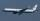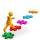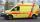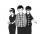Two cars

From the town A to town B started two cars. The first at 7:00 at average speed 60 km per hour, the second at 10:00 at average speed 100 km per hour. The first car will not stay in B, and on the way back meet the second car at half way from A to B. At what time in hours its will meet?

Result

t = 10:45 hSolution:

1.5 s = 60(t-7)
0.5 s = 100(t-10)

1.5s-60t = -420
0.5s-100t = -1000

s = 150
t = 434 = 10.75

Calculated by our linear equations calculator.

Leave us a comment of this math problem and its solution (i.e. if it is still somewhat unclear...):Be the first to comment!To solve this verbal math problem are needed these knowledge from mathematics:

Do you have a linear equation or system of equations and looking for its solution? Or do you have quadratic equation? Do you want to convert length units?

Next similar math problems:

1. Warehouse carsFrom the warehouse started truck at speed 40km/h. After 1hour 30mins started from the same place same direction a car at speed 70 km/h. For how long and at what distance from the warehouse overtake a truck?
2. Two planesTwo planes flying from airports A and B, 420 km distant against each other. Plane from A took off 15 minutes later and flies at an average speed of 40 km/h higher than the plane from B. Determine the average speed of this two aircraft if you know that it
3. Three workshopsThere are 2743 people working in three workshops. In the second workshop works 140 people more than in the first and in third works 4.2 times more than the second one. How many people work in each workshop?
4. Journey to schoolOn the way to school I went steady step. In the first half of the journey, I counted every second step, in the second half every third. How many steps I do when I went to school if I counted double step are 250 more than three steps?
5. SchoolsThree schools are attended by 678 pupils. To the first attend 21 students more and to the third 108 fewer students than to second school. How many students attend the schools?
6. Boxes200 boxes have been straightened in three rows. The first was 13 more than in the second, and in the second was one fifth more than in the third one. How many boxes are in each row?
7. CarCar goes some distance in 3 hours and 20 minutes. If it increase speed by 10 km/h, i goes this distance in 2.5 hours. Calculate the distance.
8. Jane plantsJane plants flowers in the garden. If she planted 12 every hour instead of 9 flowers, she would finish with the job an hour earlier. How many flowers does she plant?
9. Ages 2A man's age is 4 times his son's age. After 5 years he will be just twice his son's age, find their ages.
10. EquationsSolve following system of equations: 6(x+7)+4(y-5)=12 2(x+y)-3(-2x+4y)=-44
11. ThreesomeDana, Dalibor and Michael have a combined 57 years. Dana is five years older than Dalibor, but Dana is five years younger than Michael. Determine how old is Dana, Dalibor and Michael.
12. Three figures - numbersThe sum of three numbers, if each is 10% larger than the previous one, is 662. Determine the figures.
13. PoojaPooja and Deepa age is 4:5, 4 years back it was 8:11. What is the age of Pooja now?
14. Father and sonWhen I was 11, my father was 35 years old. Today, my father has three times more years than me. How old is she?
15. Elimination methodSolve system of linear equations by elimination method: 5/2x + 3/5y= 4/15 1/2x + 2/5y= 2/15
16. CarlaCarla is 5 years old and Jim is 13 years younger than Peter. One year ago, Peter’s age was twice the sum of Carla’s and Jim’s age. Find the present age of each one of them.
17. PearsAndrew, Lenka and Rasto have together 232 pears. Lenka has 28 more than Rasto and Rasto pears have 96 more than Andrew. Determine how much each of them has pears.